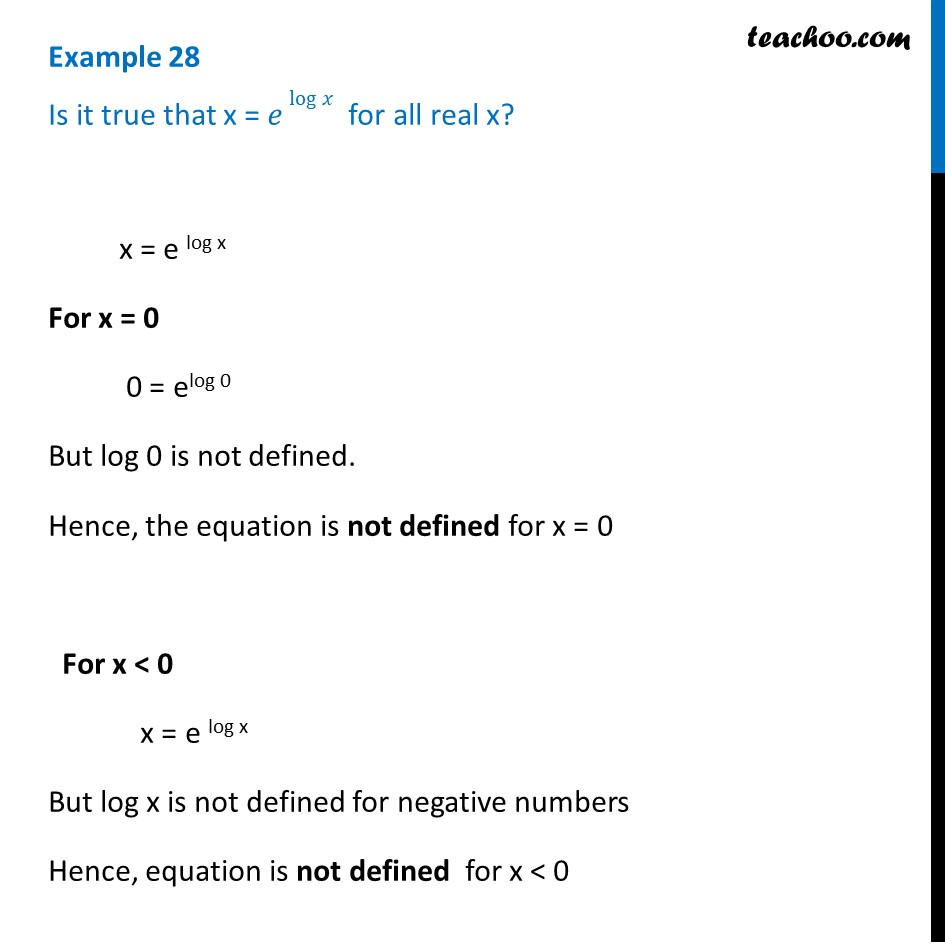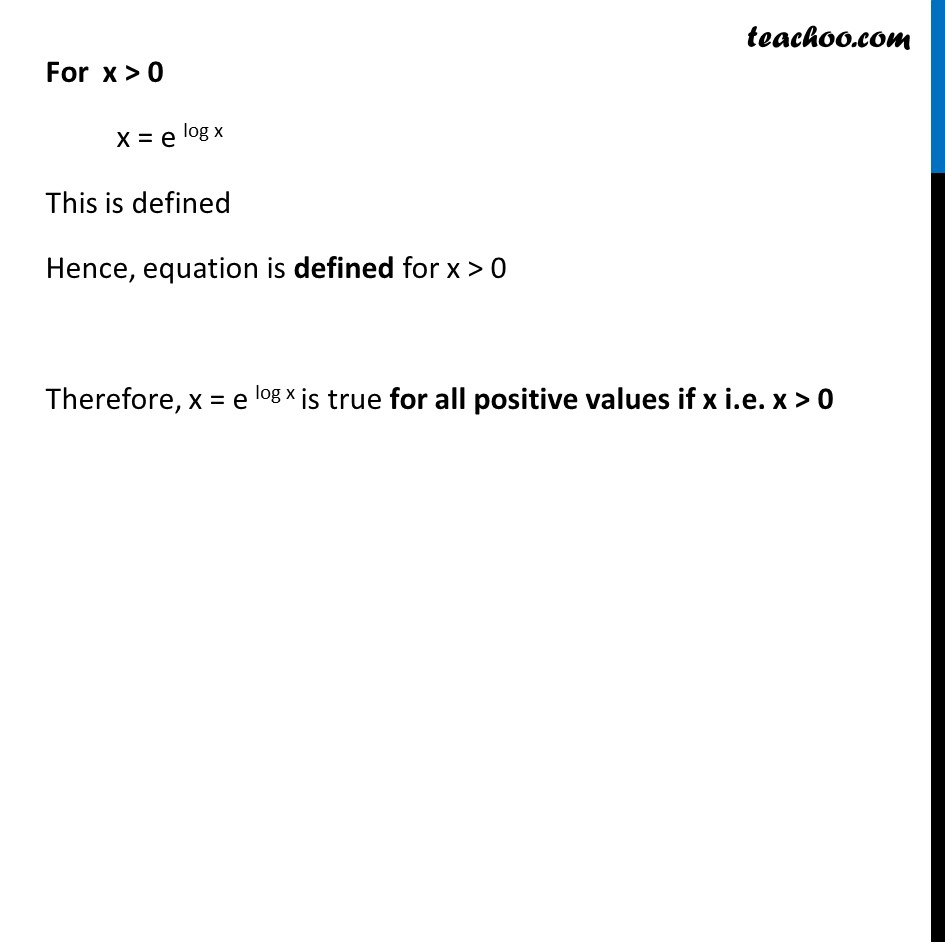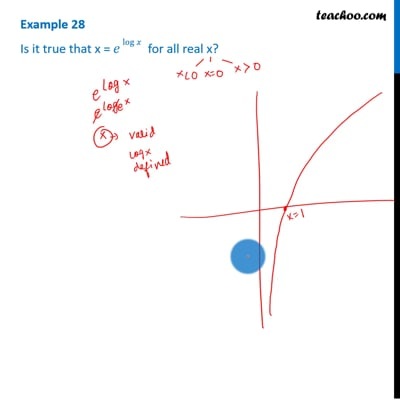Finding derivative of Exponential & logarithm functions

Chapter 5 Class 12 Continuity and Differentiability
Concept wiseThis video is only available for Teachoo black users

Introducing your new favourite teacher - Teachoo Black, at only ₹83 per month

### Transcript

Example 28 Is it true that x = 𝑒 log⁡𝑥 for all real x? x = e log x For x = 0 0 = elog 0 But log 0 is not defined. Hence, the equation is not defined for x = 0 For x < 0 x = e log x But log x is not defined for negative numbers Hence, equation is not defined for x < 0 For x > 0 x = e log x This is defined Hence, equation is defined for x > 0 Therefore, x = e log x is true for all positive values if x i.e. x > 0